# 针对交易的组合数学和概率论（第二部分）：通用分形

11 十月 2021, 10:08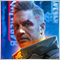0
799

### 评估在交易中运用分形的可能性

1. 具有对称边界的分形
2. 具有非对称边界的分形
3. 具有上边界或下边界的分形

• 加速模拟交易，能够评估各种场景的可能性，同时考虑存款限制（因为下边界能表征无法进一步交易的存款底线）
• 分形内平均步阶数的估算（例如，您可以估算在我们获得所需的利润或亏损之前我们平均有多少订单）
• 评估每个步阶的总平均值（例如，您可以根据一笔较小持仓的统计数据来计算平均持仓时间，即较小止损点数或价格差异的持仓
• 基于单边分形的盈利能力评估
• 其它能力

### 构建通用分形的理论基础

1. m = n & [ m > s & n > s ]
2. ( m > n || n > m )  & [ m > s & n > s ]
3. ( m > S && n <= S ) || ( n > S && m <= S )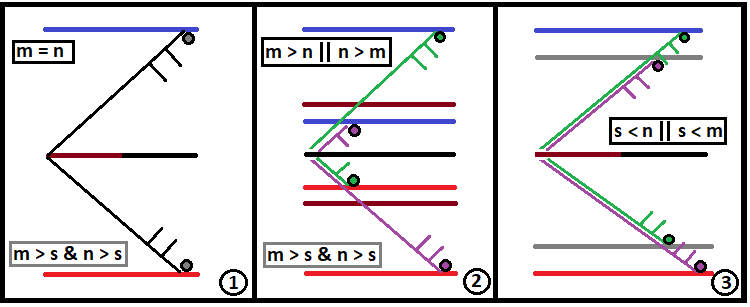• Half - 通道宽度的一半
• Middle - 对应于中线的 “U” 值

• Half = ( n + m ) / 2

1. n >= m
2. n < m

1. Middle = Half - m
2. Middle = - ( Half - n )

• double Fractal(double Half, double Middle, int m, int n, int s,double p,int S, int U, double P)

1. Half - 通道宽度的一半
2. Middle - 对应于中线的 “U” 值
3. m - 到下边界的步阶数
4. n - 到上边界的步阶数
5. s - 单个链在任何方向上允许的最大步阶数

• S - 当前概率链中累积的步阶数； 要被传递到下一个分形层
• U - 链起点和终点之间的当前距离； 传递到下一个分形层
• P - 基于伯努利规划案的完整概率链的累积乘积； 需要传递到下一个分形层

• S = 0 (既然是起点，所以还没有步阶)
• U = 0 (出于同样的原因)
• P = 1 (因为它是一个零链，所有接下来的步阶应构成一个完整的组)

• f = u + d  — 它是未来组合树的步阶数（距离则由到分形范围最近边界的距离决定）
• s = u - d  — 最终步阶数量，以下跌段落和上涨段落表示

• s = 2*u - f

• NewU = s + U  - 我们要传递到下一个分形层的新 “U”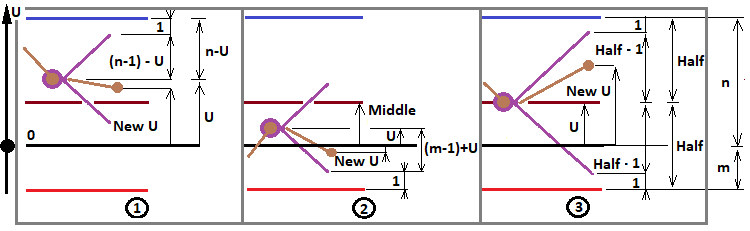1. f = ( n - 1 ) - U
2. f = ( m - 1 ) + U
3. f = Half - 1

1. U > Middle
2. U < Middle
3. U = Middle

• NewP = P * C(f,i) * Pow(p,i) * Pow(1-p,f-i)  — 我们的新链概率 “P” 要被传递到下一个分形层
• NewS = S + f = S + (floor(Mid) - 1) — 我们的新 “S” 要被传递到下一个分形层

1. U = n - 1
2. U = - ( m - 1 )

• NewU = U - 1
• NewP = P * p
• NewS = S + 1

• NewU = U + 1
• NewP = P * ( 1 - p )
• NewS = S + 1

• 分形计算跨越走廊上边界的总概率
• 分形计算跨越走廊下边界的总概率

• 分形计算跨越上边界的平均步阶数
• 分形计算跨越下边界的平均步阶数

1.  P * p * NewS
2.  P * ( 1 - p ) * NewS

1. SU - 所选概率链的最后上升步阶
2. SD - 所选概率链的最后下降步阶
3. NewSU 和 NewSD - 要传递到下一个分形层的值
4. SU + SD = S

• NewSU = SU
• NewSD = SD + 1

"U < Middle":

• NewSU = SU + 1
• NewSD = SD

1. UpperMidSDown - 到达上边界之前的总平均可能下降步阶数
2. UpperMidSUp - 在到达上边界之前可能上升的总平均步阶数
3. UpperSummProbability - 跨越上边界的概率
4. LowerMidSDown - 到达下边界之前的总平均可能下降步阶数
5. LowerMidSUp - 到达下边界之前的总平均可能上升步阶数
6. LowerSummProbability - 跨越下边界的概率

### 编写实现通用分形的代码

```Container StartFractal(int m, int n, int s,double p)//preparing all variables and starting the fractal
{
int Minimum;
if ( m <= n ) Minimum=m;
else Minimum=n;
double Middle;
if ( n >= m ) Middle = (m+n)/2.0 - Minimum;
else Middle = -((m+n)/2.0 - Minimum);
double Half = (m+n)/2.0;
return Fractal(Half,Middle,m,n,s,p,0,0,0,1.0);
}
```

```struct Container//a container for collecting all the necessary data about the fractal
{
//values to be summed, for the upper bound
double UpperMidSUp;//the sum of probabilities multiplied by the number of steps up of a specific chain (to cross the upper bound)
double UpperMidSDown;//the sum of probabilities multiplied by the number of steps down of a specific chain (to cross the upper bound
double UpperSummProbability;//the sum of the probabilities (to cross the upper border)
//values to be summed, for the lower border
double LowerMidSUp;//the sum of probabilities multiplied by the number of steps up of a specific chain (to cross the lower border)
double LowerMidSDown;//the sum of probabilities multiplied by the number of steps down of a specific chain (to cross the lower border)
double LowerSummProbability;//the sum of the probabilities (to cross the lower border)

Container()//default constructor
{
UpperMidSUp=0.0;
UpperMidSDown=0.0;
UpperSummProbability=0.0;
LowerMidSUp=0.0;
LowerMidSDown=0.0;
LowerSummProbability=0.0;
}

//
{
c0.UpperMidSUp=c0.UpperMidSUp+c1.UpperMidSUp;
c0.UpperMidSDown=c0.UpperMidSDown+c1.UpperMidSDown;
c0.UpperSummProbability=c0.UpperSummProbability+c1.UpperSummProbability;
c0.LowerMidSUp=c0.LowerMidSUp+c1.LowerMidSUp;
c0.LowerMidSDown=c0.LowerMidSDown+c1.LowerMidSDown;
c0.LowerSummProbability=c0.LowerSummProbability+c1.LowerSummProbability;
}
void operator+=(Container &c) { Summ(this,c); }//operator += overload
};
```

```Container Fractal(double Half, double Middle, int m, int n, int s,double p,int SU,int SD, int U, double P)//Fractal
{
Container C;
///to pass to the next fractal level
int NewU;
int NewSU;
int NewSD;
double NewP;
///

if ( U > Middle && SU + SD < s )//case 1
{
if ( (n-1) - U > 0 )
{
for ( int u=0 ; u <= (n-1) - U; u++ )
{
NewU = -(n-1) + 2*u + 2*U;
NewP = P * (Factorial((n-1) - U)/(Factorial(u)*Factorial((n-1) - U - u))) * pow(p,u)*pow(1.0-p,(n-1) - U - u);
NewSU = SU + u;
NewSD = SD + ((n-1) - U - u);
C+=Fractal(Half,Middle,m,n,s,p,NewSU,NewSD,NewU,NewP);
}
}
if ( (n-1) - U == 0 )
{
NewU = U - 1;
NewP = P * (1.0 - p);
NewSU = SU;
NewSD = SD + 1;
Container ct;

ct.UpperMidSDown=P*p*SD;
ct.UpperMidSUp=P*p*(SU+1);
ct.UpperSummProbability=P*p;

C+=ct;
C+=Fractal(Half,Middle,m,n,s,p,NewSU,NewSD,NewU,NewP);
}
}

if ( U < Middle && SU + SD < s )//case 2
{
if ( (m-1) + U > 0 )
{
for ( int u=0 ; u <= (m-1) + U; u++ )
{
NewU = -(m-1) + 2*u;
NewP = P * (Factorial((m-1) + U)/(Factorial(u)*Factorial((m-1) + U - u))) * pow(p,u)*pow(1.0-p,(m-1) + U - u);
NewSU = SU + u;
NewSD = SD + ((m-1) + U - u);
C+=Fractal(Half,Middle,m,n,s,p,NewSU,NewSD,NewU,NewP);
}
}
if ( (m-1) + U == 0 )
{
NewU = U + 1;
NewP = P * p;
NewSU = SU + 1;
NewSD = SD;
Container ct;

ct.LowerMidSDown=P*(1.0 - p)*(SD+1);
ct.LowerMidSUp=P*(1.0 - p)*SU;
ct.LowerSummProbability=P*(1.0 - p);

C+=ct;
C+=Fractal(Half,Middle,m,n,s,p,NewSU,NewSD,NewU,NewP);
}
}

if ( U == Middle && SU + SD < s )//case 3
{
if ( int(MathFloor(Half))-1 > 0 )
{
for ( int u=0 ; u <= int(MathFloor(Half))-1; u++ )
{
NewU = -(int(MathFloor(Half))-1) + 2*u + U;
NewP = P * (Factorial(int(MathFloor(Half))-1)/(Factorial(u)*Factorial(int(MathFloor(Half))-1 - u))) * pow(p,u)*pow(1.0-p,int(MathFloor(Half))-1 - u);
NewSU = SU + u;
NewSD = SD + (int(MathFloor(Half))-1 - u);
C+=Fractal(Half,Middle,m,n,s,p,NewSU,NewSD,NewU,NewP);
}
}
}

return C;
}
```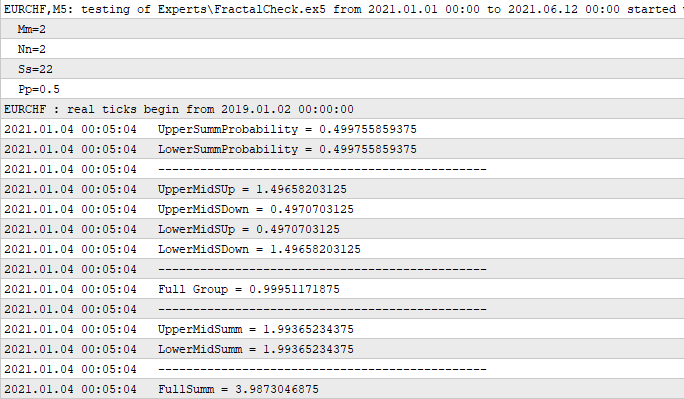### 基于对称分形推导第一个公式

• P[n] = P[n-1] * 2  - 是新走廊宽度的递推式表达式，基于前一个较小走廊的宽度，新走廊由此组成
• S[n] = S[n-1] * 4 - 是计算新走廊平均步阶数的递归表达式，由较小走廊的平均值表示

• P[n] = 2^n , n = 0 ... + infinity
• S[n] = 4^n = (2^2)^n = (2^n)^2 = P[n]^2

• P[n] = 3^n , n = 0 ... + infinity
• S[n] = 9^n = (3^2)^n = (3^n)^2 = P[n]^2

• S = S0 * K^2 - 新走廊的平均步阶数
• T = S * T0 - 新走廊的平均生存期
• T = T0 * K^2- 依据另一条走廊的平均生存期表示的新走廊的平均生存期（假设 S0 = 1 ）
• S0 - 旧走廊的平均步阶数
• T0 - 旧走廊一个步阶的平均生存期
• P = K * P0  --> K = P/P0 - 新走廊比旧走廊的放大倍数
• P - 新走廊的宽度
• P0 - 旧走廊的宽度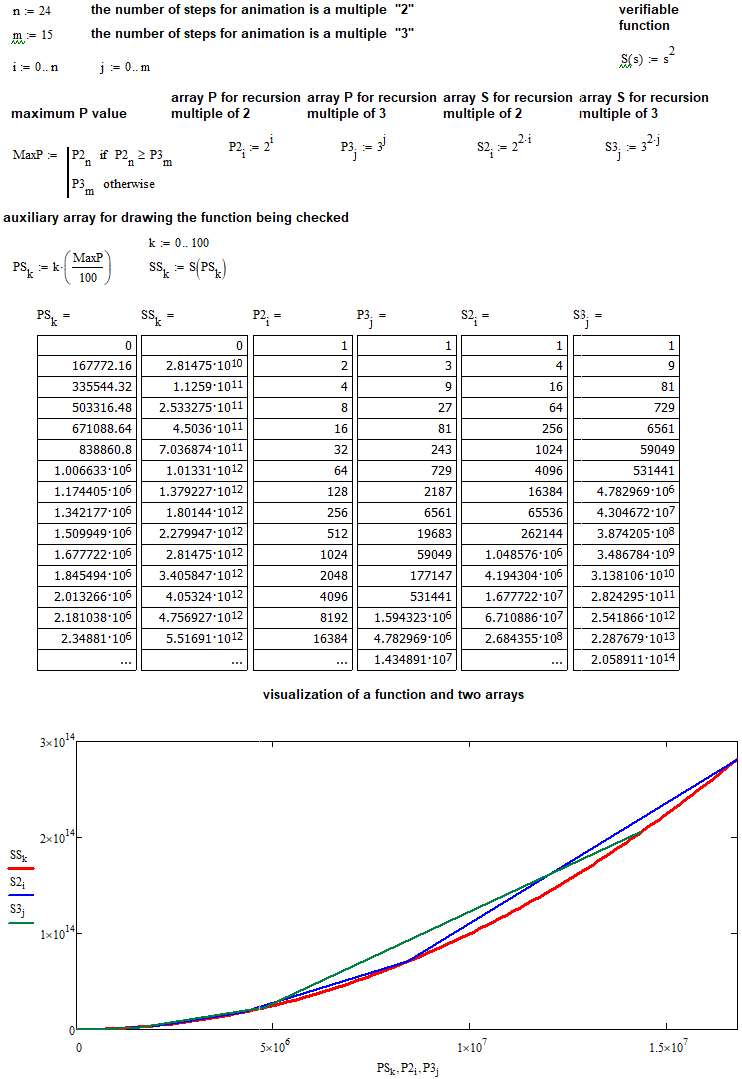### 评估所有正值和实数型参数的导出公式的性能

• T = T0 * N^2 ---> T0 = T / N^2
• T - 是我们走廊的生存期，我们已知其平均生存期
• T0 - 是一个较小走廊的平均生存期，由我们的走廊组成

• d = P / N

• Smin = MathFloor( K * P / d ) = MathFloor( K * N )
• Lim( N --> +infinity ) [ K * N/MathFloor( K * N ) ] = 1

• Smax = MathFloor( K * P / d ) + 1 =  MathFloor( K * N ) + 1 = Smin + 1

• T1 =( T0 * Smin^2 + T0 * Smax^2 ) / 2 =  T0 *( Smin^2 + Smax^2 ) / 2
• T1 - 我们需要确定的走廊的平均生存期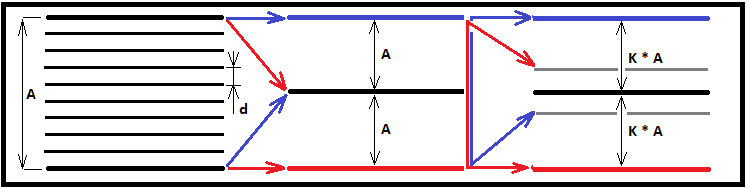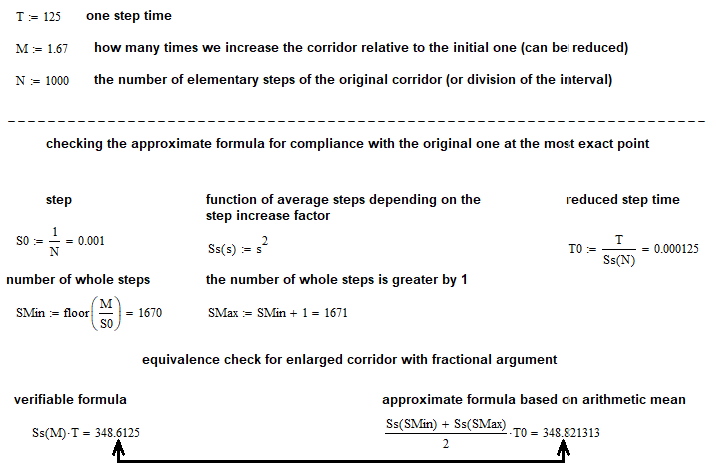• Lim( N --> +infinity ) [  (T0 *( Smin^2 + Smax^2 ) / 2) / ( T * K^2 )  ] = 1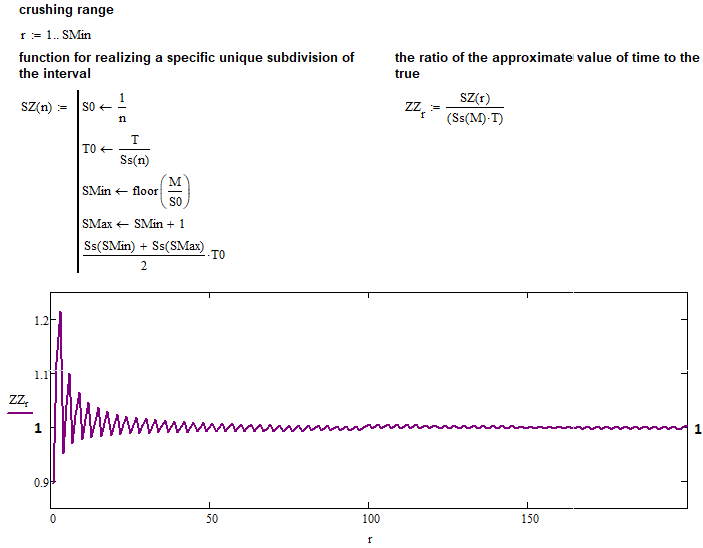### 自通用分形发展而来的高级分形

• s = 22 , FullSumm = 2.868 , UpperSummProbability = 0.831
• s = 32 , FullSumm = 3.618 , UpperSummProbability = 0.860
• s = 42 , FullSumm = 4.262 , UpperSummProbability = 0.877
• s = 45 , FullSumm = 4.499 , UpperSummProbability = 0.882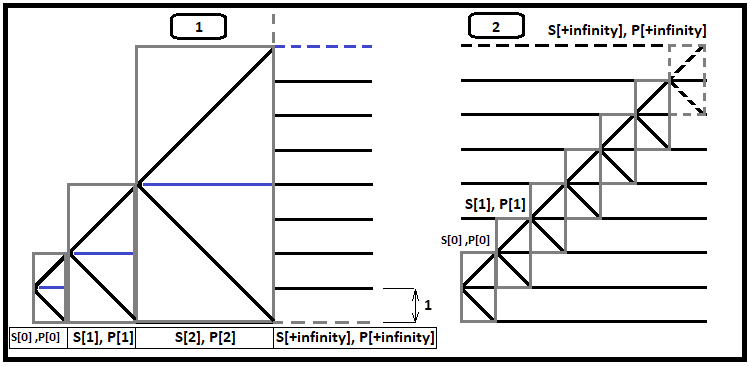1. 价格到达箱子的上边界
2. 价格触及箱子的下边界

• PUp = PDown = P - 示意触及分形上限和下限的概率对于所有嵌套分形的所有边界的概率相等的比率
• P[j] = 0.5^(j+1) , j = 0 ... + infinity- 链 j 发生的概率
• S[i] = S[i-1] + P[i] * ( S[i-1]/P[i-1] + F(D[i]) ),  i = 1... + infinity - 一个递归公式，计算所有分形层的总平均可能步阶数（而 S = 1*0.5 = 0.5
• F(K) = K^2 - 我们计算平均步阶数的导出公式
• D(i) = 2^i - 下一个分形层容纳多少步阶
• S[i-1]/P[i-1] - 其余未计分支的平均步阶数，前提是当前分支已经发生（因为除了当前嵌套分形之外，还需要考虑之前发生的所有步阶）

• S[j] = S[j-1] + P[j] * ( S[i-1]/P[i-1] + F(1) ) - 一个递归公式，计算所有分形层的总平均可能步阶数（而 S = 1*0.5 = 0.5）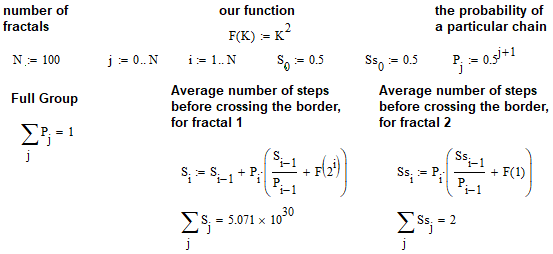### 总结成果

• 为构建通用分形定义了清晰的数学规则
• 根据所界定的数学原理创建了一套有效的 MQL5 风格的代码
• 运用算法，得到首个计算任意走廊生存期的公式
• 针对所有可能的情况，利用编程测试和验证公式
• 基于所获公式得到一种更快速的分形新种类
• 结果令我们能够加快计算，并判断通用分形无法判别的情况
• 触及使用高级分形解决掉期交易问题的特例
• 获得进一步发展理论的工具

1. S = K^2 - 新走廊的平均步阶数，基于一个步阶与另一条走廊相等的事实
2. T = S * T0 =  T0 * K^2- 未知走廊的平均生存期
3. T0 - 已知走廊的平均生存期
4. P = K * P0  --> K = P/P0 - 已知走廊比未知走廊大多少倍
5. P - 生存期不明的走廊宽度
6. P0 - 已知走廊的宽度

• T0, P0, P

• 好吧，并非总能收集到统计数据，因为并非所有金融品种都拥有进行评估所需的足够交易历史。 这对于大型走廊来说是不可能的，在于总能从未跨越边界的走廊里选择其一。 使用较小宽度的走廊和公式，我们便可以获得统计中无法获得的数据。 此外，这种数学方法能为您提供无与伦比的准确性和灵活性。 还有更多其它优点。

### 参考

• #### 针对交易的组合数学和概率论（第一部分）：基础知识

Materials.zip (270.97 KB)

#### 该作者的其他文章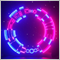针对交易的组合数学和概率论（第三部分）：第一个数学模型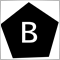更好的程序员（第 02 部分）：停止做这 5 件事变为一名成功的 MQL5 程序员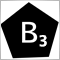更好的程序员（第 03 部分）：放弃做这 5 件事成为一名成功的 MQL5 程序员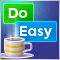DoEasy 函数库中的图形（第八十一部分）：将图形集成到函数库对象之中